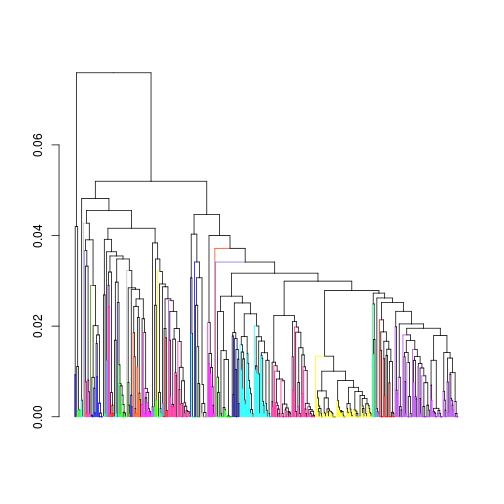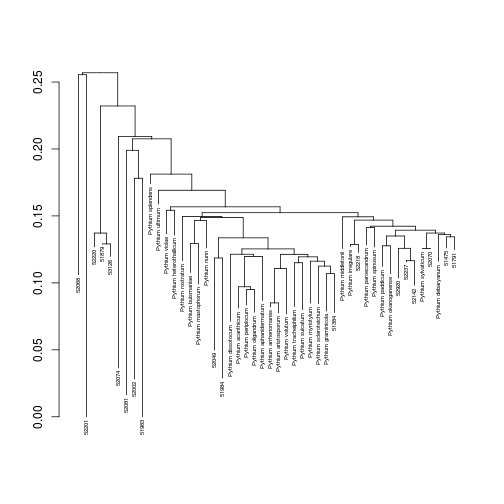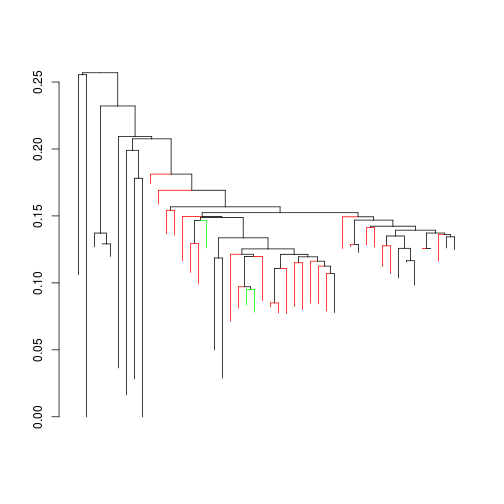The objective of this workflow is to detect known Pythium species in several samples collected from soybean fields in Pennsylvania. Pythium is a eukaryotic microorganism that can help or harm plants depending on the species. Many Pythium species cause root rot in specific plants. However, some species of Pythium are used as biological control agents to prevent the growth of pathogens on crops. This workflow parallels an analysis of the same datasets performed by Coffua et al. in the journal Plant Disease (2016).

Here the Cytochrome c oxidase subunit 1 (COI) gene is used as a phylogenetic marker to identify species. The COI gene is part of the mitochondrial genome of all eukaryotes. In part #1 of this workflow, the goal is to design optimal primers to differentiate all of the Pythium species using the COI gene. The first step is to download the COI gene sequences of known Pythium species from the Internet and import them into a sequence database, as shown below.

``````# all paths are relative to the installed datasets
data_dir <- system.file("extdata", package="BigBioSeqData")

library(DECIPHER)
``````
``````## Loading required package: Biostrings
``````
``````## Loading required package: BiocGenerics
``````
``````## Loading required package: parallel
``````
``````##
## Attaching package: 'BiocGenerics'
``````
``````## The following objects are masked from 'package:parallel':
##
##     clusterApply, clusterApplyLB, clusterCall, clusterEvalQ,
##     clusterExport, clusterMap, parApply, parCapply, parLapply,
##     parLapplyLB, parRapply, parSapply, parSapplyLB
``````
``````## The following objects are masked from 'package:stats':
##
``````
``````## The following objects are masked from 'package:base':
##
##     Filter, Find, Map, Position, Reduce, anyDuplicated, append,
##     as.data.frame, cbind, colnames, do.call, duplicated, eval,
##     evalq, get, grep, grepl, intersect, is.unsorted, lapply,
##     lengths, mapply, match, mget, order, paste, pmax, pmax.int,
##     pmin, pmin.int, rank, rbind, rownames, sapply, setdiff, sort,
##     table, tapply, union, unique, unsplit, which, which.max,
##     which.min
``````
``````## Loading required package: S4Vectors
``````
``````## Loading required package: stats4
``````
``````##
## Attaching package: 'S4Vectors'
``````
``````## The following objects are masked from 'package:base':
##
##     colMeans, colSums, expand.grid, rowMeans, rowSums
``````
``````## Loading required package: IRanges
``````
``````## Loading required package: XVector
``````
``````## Loading required package: RSQLite
``````
``````## Loading required package: DBI
``````
``````# Create a connection to an on-disk SQLite database
dbConn <- dbConnect(SQLite(),
"./COIgenes.sqlite") # path to new database file

# Import sequences from a GenBank formatted file
Seqs2DB(paste(data_dir,
"/Pythium_spp_COI.gb",
sep=""),
type="GenBank",
dbFile=dbConn,
identifier="Pythium")

# View the database table that was constructed
BrowseDB(dbConn)

# Retrieve the imported sequences
dna <- SearchDB(dbConn)
dna

# Align the sequences based on their translations
DNA <- AlignTranslation(dna)
DNA

# Display the sequences in a web browser
BrowseSeqs(DNA)
# show differences with the first sequence
BrowseSeqs(DNA, highlight=1)
# show differences with the consensus sequence
BrowseSeqs(DNA, highlight=0)
# change the degree of consensus
BrowseSeqs(DNA, highlight=0, threshold=0.2)

# note the pattern common to most sequences
pattern <- DNAStringSet("TAGATTTAGCWATTTTTAGTTTACA")
BrowseSeqs(DNA,
patterns=pattern)

# The protein sequences are very similar
AA <- AlignTranslation(dna, asAAStringSet=TRUE)
BrowseSeqs(AA, highlight=1)

# Choose a reference for frameshift correction
REF <- translate(dna) # sequence #11

# Correct the frameshift in sequence #12
correct <- CorrectFrameshifts(myXStringSet=dna,
myAAStringSet=REF,
type="both")
correct
dna <- correct\$sequence

# Sequence #11 is now identical to #12
DNA <- AlignTranslation(dna)
BrowseSeqs(DNA, highlight=11)

# Identify clusters for primer design
d <- DistanceMatrix(DNA)
dim(d) # a symmetric matrix
c <- IdClusters(d,
method="UPGMA",
cutoff=0.05,
show=TRUE)
````````````head(c) # cluster numbers

# Identify sequences by cluster name in the database
c\$cluster,
sep="")),
dbConn)
BrowseDB(dbConn)

# Design primers for next-generation sequencing
primers <- DesignSignatures(dbConn,
type="sequence",
resolution=5,
levels=5,
minProductSize=400,
maxProductSize=800,
annealingTemp=55,
maxPermutations=8)
primers[1,] # the top scoring primer set

# Highlight the primers' target sites
BrowseSeqs(DNA,
patterns=c(DNAStringSet(primers[1, 1]),
reverseComplement(DNAStringSet(primers[1, 2]))))
``````

Part #2 of the workflow uses sequences of the COI gene that were obtained from several locations in Pennsylvania. These DNA sequences are stored in FASTQ format along with their corresponding quality scores. After importing, the first step is to trim the sequences so that only the high quality center region remains. The subset of sequences that might belong to Pythium species will be identified by the presence of a conserved region common all Pythium. This analysis will be performed in batches so that all of the sequences do not need to fit in memory simultaneously.

``````# Import from the compressed FASTQ sequence files
path <- paste(data_dir,
"/FASTQ/",
sep="")
files <- list.files(path)
samples <- substring(files,
first=1,
last=nchar(files) - 6)
for (i in seq_along(files)) {
cat(samples[i], ":\n", sep="")
Seqs2DB(paste(path, files[i], sep=""),
type="FASTQ",
dbFile=dbConn,
identifier=samples[i],
}

# Function for determining boundaries
# of the high-quality central region
bounds <- function(probs,
thresh=0.001,
width=21) {

# Calculate a moving average
probs,
probs <- filter(probs,
rep(1/width, width))

# Find region above the threshold
w <- which(probs < thresh) - padding
if (length(w)==0)
w <- NA

return(c(w, w[length(w)]))
}

# Trim the sequences by quality and identify
# the subset belonging to the Pythium genus
nSeqs <- SearchDB(dbConn,
count=TRUE,
verbose=FALSE)
offset <- 0
ends <- starts <- counts <- integer(nSeqs)
fprimer <- DNAString("TCAWCWMGATGGCTTTTTTCAAC")
rprimer <- DNAString("")
pBar <- txtProgressBar(max=nSeqs, style=3)
while (offset < nSeqs) {
# Select a batch of sequences
dna <- SearchDB(dbConn,
type="QualityScaledXStringSet",
limit=paste(offset, 1e4, sep=","),
verbose=FALSE)

# Convert quality scores to error probabilities
probs <- as(quality(dna), "NumericList")
endpoints <- sapply(probs, bounds)

# Store the results for later use
index <- (offset + 1):(offset + length(dna))
starts[index] <- ifelse(endpoints[1,] >= 38L,
endpoints[1,],
38L) # first base after the forward primer
ends[index] <- ifelse(endpoints[2,] >= starts[index],
endpoints[2,],
starts[index] - 1L) # no high quality bases

# Find the pattern expected in Pythium sequences
counts[index] <- vcountPattern(pattern[],
subject=dna,
max.mismatch=4,
with.indels=TRUE,
fixed="subject") # allow ambiguities

offset <- offset + 1e4
setTxtProgressBar(pBar,
ifelse(offset > nSeqs, nSeqs, offset))
}

# Add the results to new columns in the database
results <- data.frame(start=starts,
end=ends,
count=counts)
dbFile=dbConn,
verbose=FALSE)
BrowseDB(dbConn,
limit=1000)

# Cluster the reads in each sample by percent identity
for (i in seq_along(samples)) {
cat(samples[i])

# Select moderately long sequences
dna <- SearchDB(dbConn,
identifier=samples[i],
clause="count > 0 and
(end - start + 1) >= 100",
verbose=FALSE)

cat(":", length(dna), "sequences")

# Trim the sequences to the high-quality region
index <- as.numeric(names(dna))
dna <- subseq(dna,
start=starts[index],
end=ends[index])

# Cluster the sequences without a distance matrix
clusters <- IdClusters(myXStringSet=dna,
method="inexact",
cutoff=0.03, # > 97% identity
verbose=FALSE)

# Add the cluster numbers to the database
dbFile=dbConn,
verbose=FALSE)

cat(",",
length(unique(clusters[, 1])),
"clusters\n")
}

# Now the database contains a column of clusters
BrowseDB(dbConn,
limit=1000,
clause="cluster is not NULL")
``````

In part #3 of the workflow, representatives from each sequence cluster are compared to known Pythium species. The goal of this analysis is to identify which organisms present in each sample are similar to known species. The known species are separated into two groups: those that are used as biocontrol agents (good strains) and those that are known to be plant pathogens (bad strains).

``````ids <- IdentifyByRank(dbConn,
lens <- IdLengths(dbConn,
BrowseDB(dbConn)

# separate Pythium strains into good and bad groups
biocontrol <- c('Pythium oligandrum',
'Pythium nunn',
'Pythium periplocum')
pathogen <- c('Pythium acanthicum', # strawberries:
'Pythium rostratum',
'Pythium middletonii',
'Pythium aristosporum', # grasses/cereals:
'Pythium graminicola',
'Pythium okanoganense',
'Pythium volutum',
'Pythium arrhenomanes',
'Pythium buismaniae', # flowers:
'Pythium spinosum',
'Pythium mastophorum',
'Pythium splendens',
'Pythium violae', # carrots:
'Pythium paroecandrum',
'Pythium sulcatum',
'Pythium dissotocum', # potatoes:
'Pythium scleroteichum',
'Pythium myriotylum',
'Pythium heterothallicum', # lettuce:
'Pythium tracheiphilum',
'Pythium ultimum', # multiple plants:
'Pythium irregulare',
'Pythium aphanidermatum',
'Pythium debaryanum',
'Pythium sylvaticum')

# Select the longest sequence from each species
species <- SearchDB(dbConn,
nameBy="identifier",
clause=paste("identifier in (",
paste("'",
c(biocontrol,
pathogen),
"'",
sep="",
collapse=", "),
") group by identifier
having max(bases)",
sep=""))

# Select the longest sequence in each cluster
dna <- SearchDB(dbConn,
identifier="DauphinFarm", # choose a sample
clause="cluster is not null
group by cluster
having max(end - start)")

# Trim to the high quality central region
index <- as.numeric(names(dna))
dna <- subseq(dna,
start=starts[index],
end=ends[index])

# Create a tree with known and unknown species
combined <- AlignSeqs(c(dna, species))
dists <- DistanceMatrix(combined,
verbose=FALSE,
correction="Jukes-Cantor")
tree <- IdClusters(dists,
method="NJ", # Neighbor joining
asDendrogram=TRUE,
verbose=FALSE)
plot(tree,
nodePar=list(lab.cex=0.5, pch=NA))
````````````# Color known species based on their pathogenicity
tree_colored <- dendrapply(tree,
function(x) {
if (is.leaf(x)) {
if (attr(x, "label") %in% pathogen) {
attr(x, "edgePar") <- list(col="red")
} else if (attr(x, "label") %in% biocontrol) {
attr(x, "edgePar") <- list(col="green")
}

# remove the label
attr(x, "label") <- ""
}
return(x)
})
plot(tree_colored)
````````````# Disconnect from the sequence database
dbDisconnect(dbConn)
# permanently delete the database